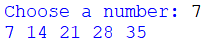## Python - Section 3 Practice Tasks

Create a program that asks the user to input a number and then prints the square of that number.

Requirements for a complete program:

1. Use only two lines.

2. Use only one print line. Include the user's number and the squared number in the print line.

Remember: Break up variables in a print line by using commas or plus signs between each part of the "sentence"

Example solution:## X

Create a program that asks the user to input two numbers. Multiply the two numbers together and print the total.

Requirements for a complete program:

1. Only three lines.

2. Use only one print line. Include the user's number and the squared number in the print line.

Remember: Break up variables in a print line by using commas or plus signs between each part of the "sentence"

Example solution:Create a program that asks the user to input their current age and then prints a guess of when they will turn 65. (Note this is more likely to be correct towards the end of the year – think about why).

You could do this in just two lines but before trying that work out on paper the steps to

calculating your own age you will turn 65. What steps did you take? Try to recreate those steps
in Python. You might need to create another variable to make it easier.

Example solution:Let the user enter a number then print the first five multiplications in its times table.

Simple example solution:Better example solution: